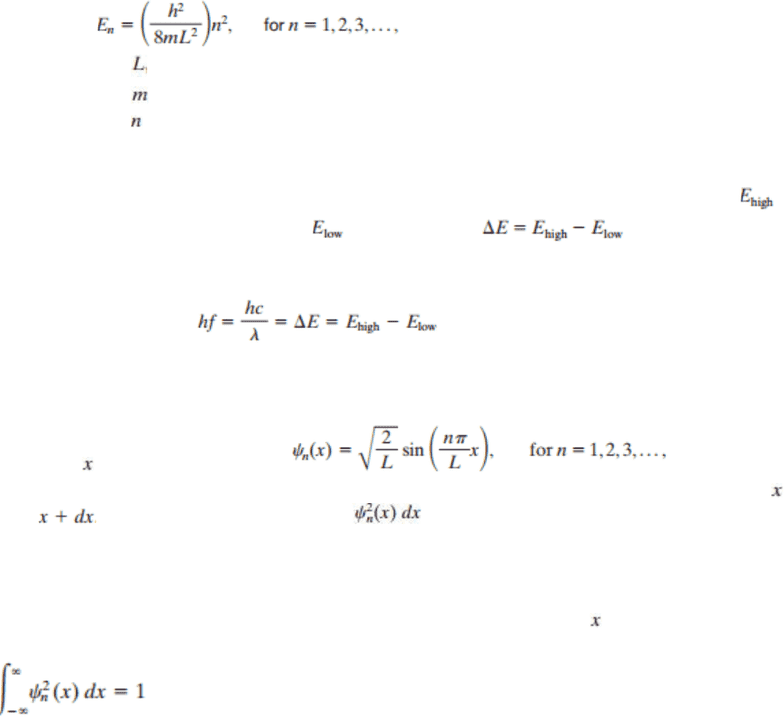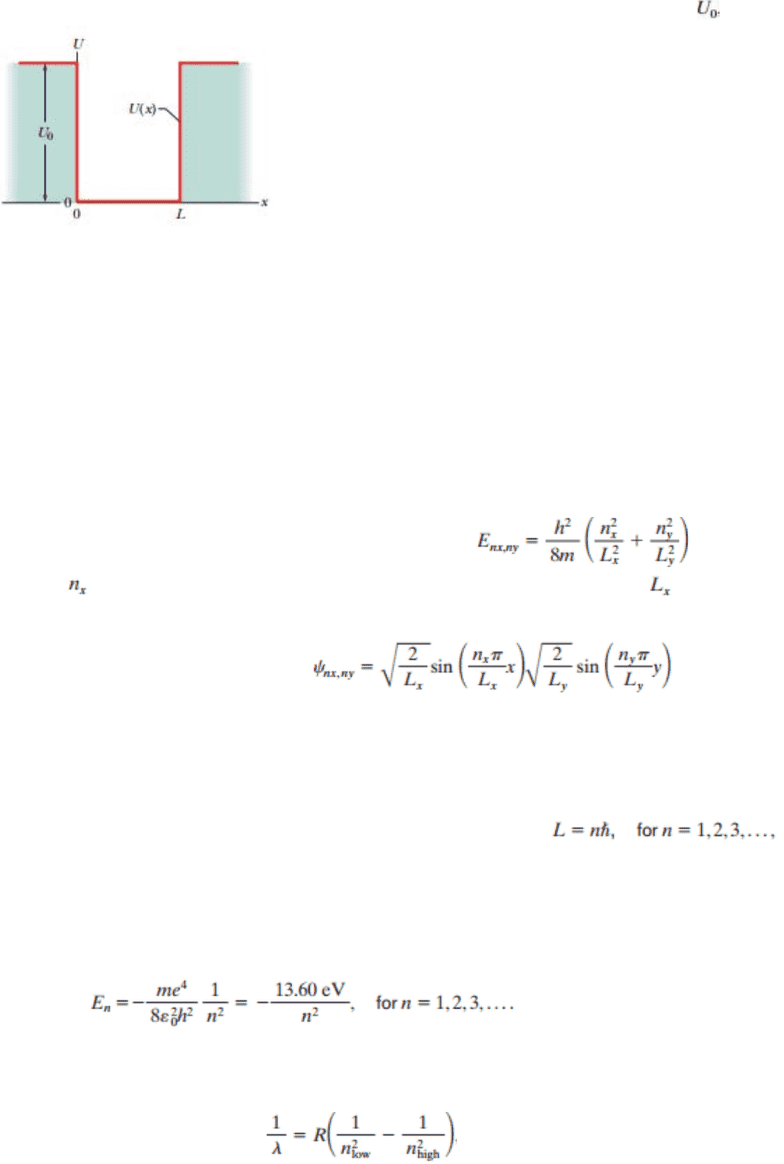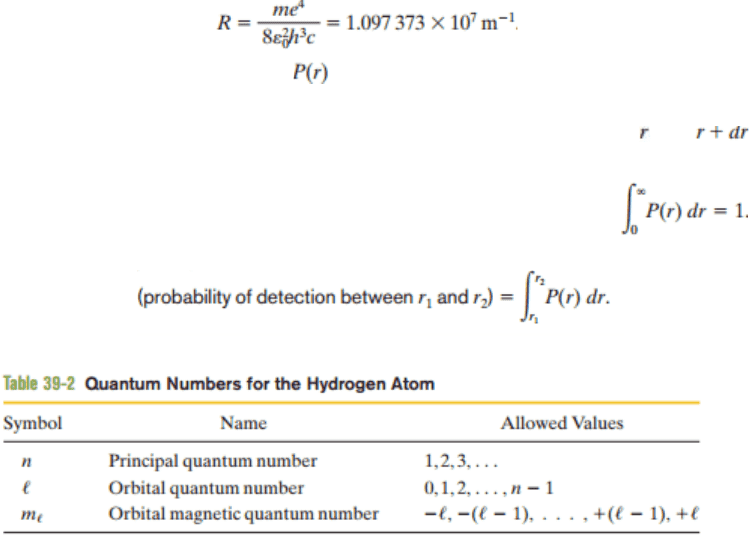Textbook Notes (270,000)
CA (160,000)
UTSG (10,000)
Physics (80)
all (30)
Study Guide

# Textbook Guide Physics: Bohr Model, Magnetic Quantum Number, Correspondence Principle

Department
Physics
Course Code
PHY131H1
Professor
all

This preview shows pages 1-3. to view the full 9 pages of the document.Chapter 39 More About Matter Waves
39-1 Energies of a Trapped Electron
The confinement principle states that confinement of any type of wave leads to
quantization, which means that the wave is restricted to discrete states with certain
energies. States with intermediate energies are not allowed within confinement.
o Electrons confined to an infinitely deep potential energy well or infinite
potential well can exist in only certain discrete states. If the well is one-
dimensional, the energies associated with these quantum state are expressed
as:
represents the length of the well.
represents the electron mass.
represents the quantum number.
The lowest energy is given by 1, not 0.
The electron can jump from one quantum state to another state only if its
energy change is equal to the difference between the higher energy ( )
to the lower energy ( ) expressed as
If change occurs by photon absorption or emission, the energy of
the photon must be equal to the change in the electron’s energy:
39-2 Wave Functions of a Trapped Electron
The wave function for an electron in an infinite one-dimensional potential energy well
along an axis is expressed as
The probability that an electron will be detected in the interval between the coordinates
and can be given by the product
The correspondence principle states that at large enough quantum numbers, the
predictions of quantum physics will coincide smoothly with the predictions of classical
physics.
If the probability density of an electron is integrated over the entire axis, the total
probability must be equal to 1; the aforementioned can be expressed as:
o Using this equation to evaluate the amplitude of a wave function is referred to as
normalizing the wave function. Thus, the equation is also referred to as the
normalization equation.
o Confined systems in quantum physics require a state of minimum energy
referred to as the zero-point energy that cannot be a quantum number of zero.
39-3 An Electron in a Finite Well

Only pages 1-3 are available for preview. Some parts have been intentionally blurred.The wave function for an electron in a finite, one-dimensional potential well extends into
the walls, where the wave function decreases exponentially with well depth ( ).
o In comparison to the sates in an infinite well of relatively the same size, the
states in a finite well have a limited number, longer de Broglie wavelengths, and
lower energies. The infinite depth potential well is an idealization.
39-4 Two- and Three-Dimensional Electron Traps
Nanocrystallites are granules of a semiconducting powdered material that is an
effective potential well for the electrons trapped within it.
Quantum dots are artificial atoms.
Quantum corrals are artificially grouped atoms in the shape of a circle utilized to move
the atoms.
The quantized energies for an electron trapped in a two-dimensional infinite potential
well that forms are rectangular corral are express by
o represents the quantum number for the respective well width ( )
The wave functions for a matter wave/electron in a two-dimensional potential well are
given by the following expression:
39-5 The Hydrogen Atom
The hydrogen atom is a fixed potential trap with the electron moving around inside it.
The Bohr model is a planetary model in which the electron orbits the central proton with
an angular momentum that is limited to values expressed as
The quantum number in the Bohr model is incorrectly restricted to exclude 0. While the
Bohr model of the hydrogen atom successfully evaluated the energy levels for the atom
in order to explain the emission and absorption spectrum (i.e. the collection of
wavelength lines) of the atom, the model is incorrect in almost every other instance.
Schrödinger’s equation gives the correct values of angular momentum and its quantized
energies:
o The atom (or electron) can change energy only by jumping between the allowed
energies.
If the jump is by photon absorption or emission, the restriction in energy is
expressed as

Only pages 1-3 are available for preview. Some parts have been intentionally blurred.(Rydberg constant)
The radial probability density ( ) for a state of hydrogen atom is defined so that the
probability density is defined as the probability of the electron being detected
somewhere in the space between two spherical shells of the radii and that are
centered on the nucleus.
o Normalization of the statement requires the expression:
The probability that the electron will be detected between any two given radii can be
expressed as
The following are the respective quantum numbers of the Hydrogen atom:
o The orbital quantum number is a measure of the magnitude of the angular
momentum associated with the corresponding quantum state.
o The orbital magnetic quantum number is related to the orientation in space of
the angular momentum vector.
###### You're Reading a Preview

Unlock to view full version

Only pages 1-3 are available for preview. Some parts have been intentionally blurred.###### You're Reading a Preview

Unlock to view full version